Setup for 5 Player a Game of Food Chain Magnate

Originally generated on 1/10/2019 11:49:01 PM

Player Setup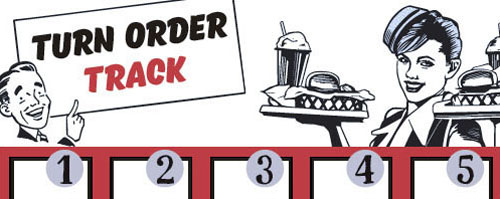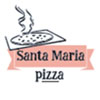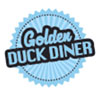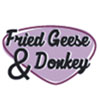Other Setup

Bank starts with \$250.00
Number of 1x Employee cards used = 3

Map (Dehydration Land)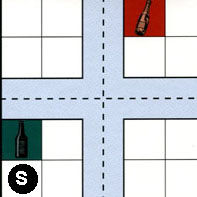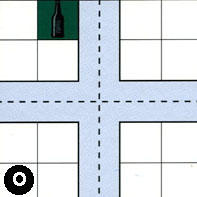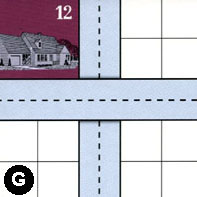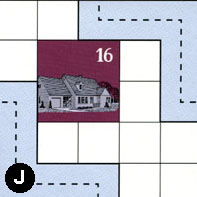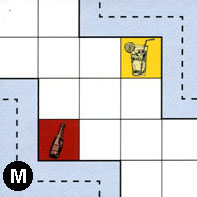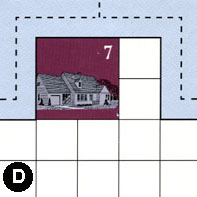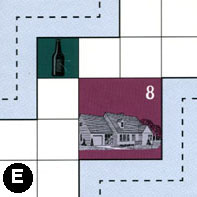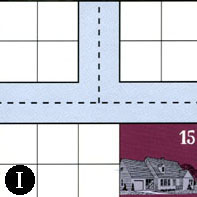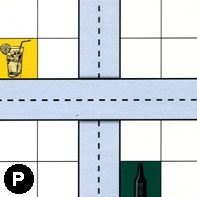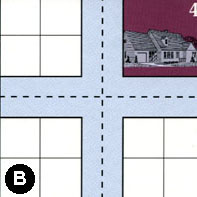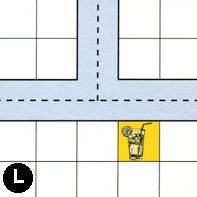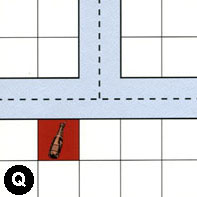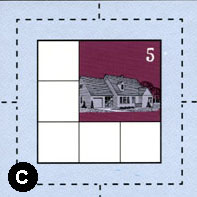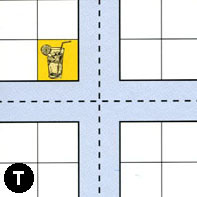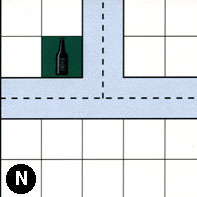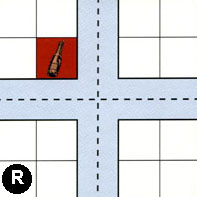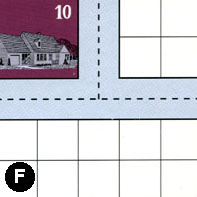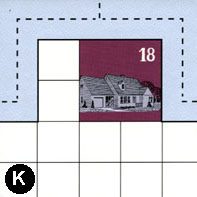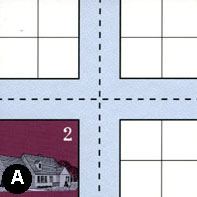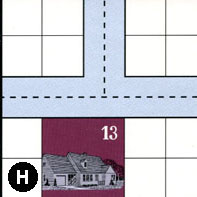Map Stats

Map Option = Dehydration Land
Total Number of Tiles = 20
Total Number of Starting Houses = 11
Total Number of Beer Spots = 5
Total Number of Soda Spots = 4
Total Number of Lemonade Spots = 4
Total Number of Drink Spots = 13
Number of independent path systems = 2
Number of independent neighborhoods = 21

Path System 1
Size = Large
Number of Tiles = 20
Number of Paths = 75
Contains Loop(s) = No
Number of Starting Houses = 12
Starting Houses Pct of Map (%) = 109
Number of Beer Spots = 5
Number of Soda Spots = 3
Number of Lemonade Spots = 4
Number of Drink Spots = 12
Drink Spots Pct of Map (%) = 92
Tile Ids = A, B, C, D, E, F, G, H, I, J, K, L, M, N, O, P, Q, R, S, T
Paths = SWN, SWE, OWN, ONE, GWE, JWS, PWE, TWS, NWN, BWE, MEN, JEN, BWN, BNE, NNE, NWE, TWN, AWN, KWN, KNE, FWE, RWN, LWN, LNE, DWE, SWS, SNS, SNE, OWE, OES, EES, DWN, SES, OWS, ONS, DNE, LWE, RWE, RNE, FWN, FNE, KWE, AWE, HWE, HWN, HNE, ANE, TNE, CWE, QWE, QWN, EWN, IWN, GNS, INE, PNS, BWS, BNS, BES, IWE, QNE, CWN, CNE, TWE, TES, TNS, ANS, AWS, AES, CES, CWS, CNS, RNS, RWS, RES What do these mean?
Starting House Ids = 0, 2, 4, 5, 7, 8, 10, 12, 13, 15, 16, 18
Path System 2
Size = Tiny
Number of Tiles = 1
Number of Paths = 1
Contains Loop(s) = No
Number of Starting Houses = 1
Starting Houses Pct of Map (%) = 9
Number of Beer Spots = 0
Number of Soda Spots = 1
Number of Lemonade Spots = 0
Number of Drink Spots = 1
Drink Spots Pct of Map (%) = 8
Tile Ids = M
Paths = MWS What do these mean?
Starting House Ids = 0

Neighborhood 1
Total Size = Small
Number of Total Spaces = 4
Number of Empty Spaces (for new houses & gardens) = 4
Number of Beer Spots = 0
Number of Soda Spots = 0
Number of Lemonade Spots = 0
Number of Drink Spots = 0
Number of Starting Houses = 0
Starting Houses Pct of Map (%) = 0
Tile Ids = S
Neighborhood 2
Total Size = Medium
Number of Total Spaces = 8
Number of Empty Spaces (for new houses & gardens) = 6
Number of Beer Spots = 1
Number of Soda Spots = 1
Number of Lemonade Spots = 0
Number of Drink Spots = 2
Number of Starting Houses = 0
Starting Houses Pct of Map (%) = 0
Tile Ids = O, S
Neighborhood 3
Total Size = Medium
Number of Total Spaces = 8
Number of Empty Spaces (for new houses & gardens) = 4
Number of Beer Spots = 0
Number of Soda Spots = 0
Number of Lemonade Spots = 0
Number of Drink Spots = 0
Number of Starting Houses = 1
Starting Houses Pct of Map (%) = 9
Tile Ids = G, O
Starting House Ids = 12
Neighborhood 4
Total Size = Large
Number of Total Spaces = 27
Number of Empty Spaces (for new houses & gardens) = 22
Number of Beer Spots = 1
Number of Soda Spots = 0
Number of Lemonade Spots = 0
Number of Drink Spots = 1
Number of Starting Houses = 1
Starting Houses Pct of Map (%) = 9
Tile Ids = B, G, J, P
Starting House Ids = 16
Neighborhood 5
Total Size = Medium
Number of Total Spaces = 19
Number of Empty Spaces (for new houses & gardens) = 17
Number of Beer Spots = 0
Number of Soda Spots = 1
Number of Lemonade Spots = 1
Number of Drink Spots = 2
Number of Starting Houses = 0
Starting Houses Pct of Map (%) = 0
Tile Ids = B, M
Neighborhood 6
Total Size = Medium
Number of Total Spaces = 24
Number of Empty Spaces (for new houses & gardens) = 19
Number of Beer Spots = 1
Number of Soda Spots = 0
Number of Lemonade Spots = 0
Number of Drink Spots = 1
Number of Starting Houses = 1
Starting Houses Pct of Map (%) = 9
Tile Ids = D, L, S
Starting House Ids = 7
Neighborhood 7
Total Size = Medium
Number of Total Spaces = 8
Number of Empty Spaces (for new houses & gardens) = 8
Number of Beer Spots = 0
Number of Soda Spots = 0
Number of Lemonade Spots = 0
Number of Drink Spots = 0
Number of Starting Houses = 0
Starting Houses Pct of Map (%) = 0
Tile Ids = O, S
Neighborhood 8
Total Size = Huge
Number of Total Spaces = 87
Number of Empty Spaces (for new houses & gardens) = 71
Number of Beer Spots = 1
Number of Soda Spots = 2
Number of Lemonade Spots = 1
Number of Drink Spots = 4
Number of Starting Houses = 3
Starting Houses Pct of Map (%) = 27
Tile Ids = A, E, F, G, I, K, L, O, Q, R, T
Starting House Ids = 8, 10, 18
Neighborhood 9
Total Size = Medium
Number of Total Spaces = 12
Number of Empty Spaces (for new houses & gardens) = 12
Number of Beer Spots = 0
Number of Soda Spots = 0
Number of Lemonade Spots = 0
Number of Drink Spots = 0
Number of Starting Houses = 0
Starting Houses Pct of Map (%) = 0
Tile Ids = G, I, P
Neighborhood 10
Total Size = Medium
Number of Total Spaces = 18
Number of Empty Spaces (for new houses & gardens) = 13
Number of Beer Spots = 0
Number of Soda Spots = 0
Number of Lemonade Spots = 1
Number of Drink Spots = 1
Number of Starting Houses = 1
Starting Houses Pct of Map (%) = 9
Tile Ids = I, P, T
Starting House Ids = 15
Neighborhood 11
Total Size = Medium
Number of Total Spaces = 16
Number of Empty Spaces (for new houses & gardens) = 15
Number of Beer Spots = 1
Number of Soda Spots = 0
Number of Lemonade Spots = 0
Number of Drink Spots = 1
Number of Starting Houses = 0
Starting Houses Pct of Map (%) = 0
Tile Ids = B, N, P, T
Neighborhood 12
Total Size = Medium
Number of Total Spaces = 8
Number of Empty Spaces (for new houses & gardens) = 4
Number of Beer Spots = 0
Number of Soda Spots = 0
Number of Lemonade Spots = 0
Number of Drink Spots = 0
Number of Starting Houses = 1
Starting Houses Pct of Map (%) = 9
Tile Ids = B, N
Starting House Ids = 4
Neighborhood 13
Total Size = Medium
Number of Total Spaces = 22
Number of Empty Spaces (for new houses & gardens) = 17
Number of Beer Spots = 0
Number of Soda Spots = 0
Number of Lemonade Spots = 1
Number of Drink Spots = 1
Number of Starting Houses = 1
Starting Houses Pct of Map (%) = 9
Tile Ids = I, P, Q, T
Starting House Ids = 15
Neighborhood 14
Total Size = Medium
Number of Total Spaces = 9
Number of Empty Spaces (for new houses & gardens) = 5
Number of Beer Spots = 0
Number of Soda Spots = 0
Number of Lemonade Spots = 0
Number of Drink Spots = 0
Number of Starting Houses = 1
Starting Houses Pct of Map (%) = 9
Tile Ids = C
Starting House Ids = 5
Neighborhood 15
Total Size = Medium
Number of Total Spaces = 8
Number of Empty Spaces (for new houses & gardens) = 8
Number of Beer Spots = 0
Number of Soda Spots = 0
Number of Lemonade Spots = 0
Number of Drink Spots = 0
Number of Starting Houses = 0
Starting Houses Pct of Map (%) = 0
Tile Ids = L, R
Neighborhood 16
Total Size = Large
Number of Total Spaces = 28
Number of Empty Spaces (for new houses & gardens) = 23
Number of Beer Spots = 0
Number of Soda Spots = 0
Number of Lemonade Spots = 1
Number of Drink Spots = 1
Number of Starting Houses = 1
Starting Houses Pct of Map (%) = 9
Tile Ids = A, H, N, T
Starting House Ids = 13
Neighborhood 17
Total Size = Small
Number of Total Spaces = 4
Number of Empty Spaces (for new houses & gardens) = 4
Number of Beer Spots = 0
Number of Soda Spots = 0
Number of Lemonade Spots = 0
Number of Drink Spots = 0
Number of Starting Houses = 0
Starting Houses Pct of Map (%) = 0
Tile Ids = R
Neighborhood 18
Total Size = Medium
Number of Total Spaces = 14
Number of Empty Spaces (for new houses & gardens) = 14
Number of Beer Spots = 0
Number of Soda Spots = 0
Number of Lemonade Spots = 0
Number of Drink Spots = 0
Number of Starting Houses = 0
Starting Houses Pct of Map (%) = 0
Tile Ids = F, R
Neighborhood 19
Total Size = Small
Number of Total Spaces = 4
Number of Empty Spaces (for new houses & gardens) = 0
Number of Beer Spots = 0
Number of Soda Spots = 0
Number of Lemonade Spots = 0
Number of Drink Spots = 0
Number of Starting Houses = 1
Starting Houses Pct of Map (%) = 9
Tile Ids = A
Starting House Ids = 2
Neighborhood 20
Total Size = Medium
Number of Total Spaces = 8
Number of Empty Spaces (for new houses & gardens) = 8
Number of Beer Spots = 0
Number of Soda Spots = 0
Number of Lemonade Spots = 0
Number of Drink Spots = 0
Number of Starting Houses = 0
Starting Houses Pct of Map (%) = 0
Tile Ids = A, H
Neighborhood 21
Total Size = Small
Number of Total Spaces = 4
Number of Empty Spaces (for new houses & gardens) = 4
Number of Beer Spots = 0
Number of Soda Spots = 0
Number of Lemonade Spots = 0
Number of Drink Spots = 0
Number of Starting Houses = 0
Starting Houses Pct of Map (%) = 0
Tile Ids = H

v2.1.5